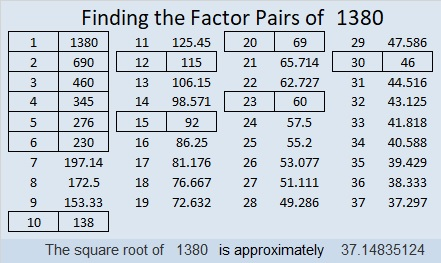# 1380 A Different Way to Look at the Logic

This puzzle looks a lot like puzzle #1378. The design is the same, but the clues are not in the same places. I made this puzzle to demonstrate that there is often more than one way to logically find all the factors of a puzzle. If it were a level 4 puzzle, the clues could be anywhere on the puzzle. But since it is a level 3 puzzle, start with the factors of 14 and 8, and then write the factors of 63 in the appropriate places. Continue with the clues in order from top to bottom until all the factors have been found.Print the puzzles or type the solution in this excel file: 10 Factors 1373-1388

Now I’ll share some information about the number 1380:

• 1380 is a composite number.
• Prime factorization: 1380 = 2 × 2 × 3 × 5 × 23, which can be written 1380 = 2² × 3 × 5 × 23
• 1380 has at least one exponent greater than 1 in its prime factorization so √1380 can be simplified. Taking the factor pair from the factor pair table below with the largest square number factor, we get √1380 = (√4)(√345) = 2√345
• The exponents in the prime factorization are 2, 1, 1, and 1. Adding one to each exponent and multiplying we get (2 + 1)(1 + 1)(1 + 1)(1 + 1) = 3 × 2 × 2 × 2 = 24. Therefore 1380 has exactly 24 factors.
• The factors of 1380 are outlined with their factor pair partners in the graphic below.There are MANY possible factor trees for 1380. Here’s one of them:1380 is the hypotenuse of a Pythagorean triple:
828-1104-1380 which is (3-4-5) times 276

This site uses Akismet to reduce spam. Learn how your comment data is processed.